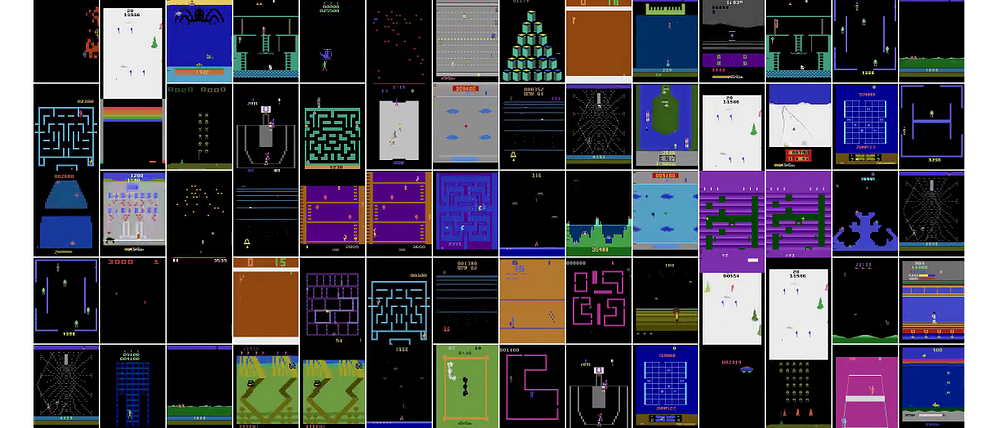# Deep Reinforcement Learning for Ping Pong

Original article can be found here (source): Deep Learning on Medium· In this post you will implement an AI program that can learn from any environment by giving rewards or punishment according to its actions respectively. Basically, we are encouraging the AI to do desired thing by giving it rewards if it acts in a desired way else punishment, how are we doing it? Follow along..!

· For the demonstration purpose we have used the game Ping Pong, provided by OpenAI’s library, as the environment for our AI. The AI gets control of one of the sliders only (green slider in our case). All the programs are done in python(Of course 😊😉).

· We will use a simple Neural Network with an input layer, a hidden layer and an output layer. It takes in input as image of current state and outputs the probability of moving the slider Up or Down and decision is made according to highest probability. This is called a policy network and has proven powerful in our case.

· By the end of this post, you’ll be able to do the following:

1. Write a Neural Network from scratch.

2. Implement a Policy Gradient with Reinforcement Learning.

3. Build an AI for Pong that can beat the computer that’s coded algorithmically to follow the ball with a speed limit for maximum speed of slider.

4. Use OpenAI gym.

# Sources:

The code and the idea are all tightly based on Andrej Karpathy’s blog post.

To follow along, you’ll need to know the following:

· Basic Python

· Neural Network design and backpropagation

· Calculus and Linear Algebra

Great! Let’s get started.

# First of all, what are Neural Networks?

To put it down simply, Neural Networks are universal function approximators that can approximate any function, meaning if we know few inputs and their corresponding outputs, we can map the inputs to the outputs and find approximately what the function was (i.e. what is the best function that describes given data). So, if the neural network is given any new input X, it can predict new output Y using this approximated function. For our case we have to find a function that takes in input an image i.e. array of float values and spits out output as correct probability of taking the slider Up (Conventionally, we have taken output as probability of slider going up, if you want the output to be probability of going down, you can do it by making changes in corresponding reward function).

Mathematically,

A single neuron (variable) does one simple task takes in the input from previous neurons, evaluates their weighted the sum and applies a sigmoid function to the sum. A layer is combination of such neurons, that take input from previous layer, and passes the output to the next layer. Together, knowing the correct weights (for finding the weighted mean) these neurons can approximate any function with mind blowing accuracy.